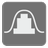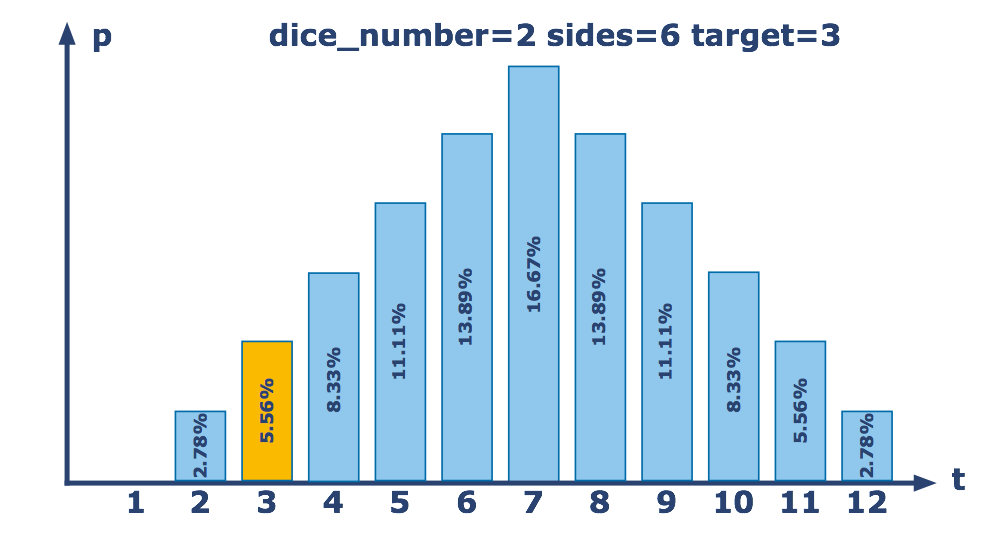Probably Dice
Moderate
English JA RU

You're on your way to a board game convention. Chances are there’ll be some stiff competition, so you decide to learn more about dice probabilities since you suspect you'll be rolling a lot of them soon.

Typically, when using multiple dice, you simply roll them and sum up all the result. To get started with your investigation of dice probability, write a function that takes the number of dice, the number of sides per die and a target number and returns the probability of getting a total roll of exactly the target value. The result should be given with four digits precision as ±0.0001. For example, if you roll 2 six-sided dice, the probability of getting exactly a 3 is 2/36 or 5.56%, which you should return as ≈0.0556.For each test, assume all the dice are the same and are numbered from 1 to the number of sides, inclusive. So a 4-sided die (D4) would have an equal chance of rolling a 1, 2, 3 or 4. A 20-sided die (D20) would have an equal chance of rolling any number from 1 to 20.

Tips: Be careful if you want to use a brute-force solution -- you could have a very, very long wait for edge cases.

Input: Three arguments. The number of dice, the number of sides per die and the target number as integers.

Output: The probability of getting exactly target number on a single roll of the given dice as a float.

Example:

```probability(2, 6, 3) == 0.0556  # 2 six-sided dice have a 5.56% chance of totalling 3
probability(2, 6, 4) == 0.0833
probability(2, 6, 7) == 0.1667
probability(2, 3, 5) == 0.2222  # 2 three-sided dice have a 22.22% chance of totalling 5
probability(2, 3, 7) == 0       # The maximum you can roll on 2 three-sided dice is 6
probability(3, 6, 7) == 0.0694
probability(10, 10, 50) == 0.0375
```

How it is used: This task illustrates some of the basics of probability. Many events can be described as the combination of other events. In this case you're combining several dice into one total to crit the Orc King for massive damage.

Preconditions:
1 ≤ dice_number ≤ 10
2 ≤ sides ≤ 20
0 ≤ target < 1000

## Become Awesome

• No Limits
• More Content16
Settings
Code:
Other:
CheckiO Extensions

CheckiO Extensions allow you to use local files to solve missions. More info in a blog post.

In order to install CheckiO client you'll need installed Python (version at least 3.8)

Install CheckiO Client first:

`pip3 install checkio_client`

`checkio --domain=py config --key=`

Sync solutions into your local folder

`checkio sync`

(in beta testing) Launch local server so your browser can use it and sync solution between local file end extension on the fly. (doesn't work for safari)

`checkio serv -d`

Alternatevly, you can install Chrome extension or FF addon

`checkio install-plugin`
`checkio install-plugin --ff`
`checkio install-plugin --chromium`

Read more here about other functionality that the checkio client provides. Feel free to submit an issue in case of any difficulties.